# Chapter 10 Congruent Triangles RD Sharma Solutions Exercise 10.1 Class 9 MathsChapter Name RD Sharma Chapter 10 Congruent Triangles Exercise 10.1 Book Name RD Sharma Mathematics for Class 10 Other Exercises Exercise 10.2Exercise 10.3Exercise 10.4Exercise 10.5Exercise 10.6 Related Study NCERT Solutions for Class 10 Maths

### Exercise 10.1 Solutions

1. In Fig. 10.22, the sides BA and CA have been produced such that : BA = AD and CA = AE. Prove that segment DE || BC.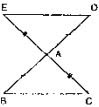Solution

Given that, the sides BA and CA have been produced such that BA = AD and CA = AE and given to prove DE || BC

Consider triangle BAC and DAE,
We have
BA = AD and CA = AE  [∵ given in the data]
And also ∠BAC = ∠DAE  [∵ vertically opposite  angles]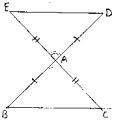So, by SAS congruence criterion, we have ΔBAC ≅ ΔDAE
⇒ BC = DE and ∠DEA = ∠BCA, ∠EDA = ∠CBA
[Corresponding parts of congruent triangles are equal]
Now, DE and BC are two lines intersected by a transversal DB such that ∠DEA = ∠BCA,
i.e., alternate angles are equal
Therefore, DE || BC

2. In a ΔPQR, if PQ = QR and L, M and N are the mid - points of the sides PQ, QR and RP respectively. Prove that : LN = MN.

Solution

Given that, in ΔPQR, PQ = QR and L, M, N are midpoints of the sides PQ, QP and RP respectively and given to prove that LN = MN
Here we can observe that PQR is and isosceles triangle
⇒ PQ = QR and ∠QPR = ∠QRP ...(1)
And also, L and M are midpoints of PQ and QR respectively
⇒ PL  = LQ = PQ/2 , QM = MR = QR/2
And also, PQ = QR
⇒ PL = LQ = QM = MR = PQ/2 = QR/2 ...(2)
Now, consider ΔLPN and ΔMRN,
LP = MR  [From (2)]
∠LPN = ∠MRN  [From  (1)]
∵∠QPR and ∠LPN and ∠QRP and ∠MRN are same
PN = NR  [∵ N is midpoint of PR]
So, by SAS congruence criterion, we have ΔLPN = ΔMRN
⇒ LN = MN
[∵ Corresponding parts of congruent triangles are equal]

3. In Fig. 10.23, PQRS is a square and SRT is an equilateral triangle. Prove that
(i) PT = QT
(ii) ∠TQR = 15°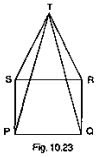Solution

Given that PQRS is a square and SRT is an equilateral triangle. And given to prove that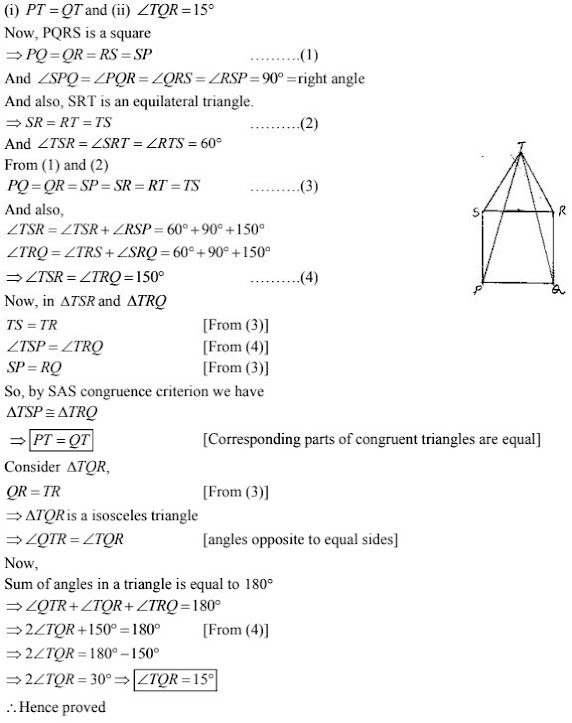4. Prove that the medians of an equilateral triangle are equal.
Solution
Given to prove that the medians of an equilateral triangle are equal
Median : The line joining the vertex and midpoint o opposite side.
Now, consider an equilateral triangle ABC
Let D, E, F are midpoints of BC, CA and AB.
Then, AD, BE and CF are medians of ΔABC.
Now,
D is midpoint of BC ⇒ BD = DC = BC/2
Similarly, CE = EA = AC/2
AF = FB = AB/2
Since, ΔABC is an equilateral triangle
⇒ AB = BC = CA  ...(1)
⇒ BD = DC = CE = EA = AF = FB = BC/2 = AC/2 = AB/2  ...(2)
And also, ∠ABC = ∠BCA = ∠CAB = 60°
Now, consider ΔABD and ΔBCE
AB = BC  [From (1)]
BD = CE  [From (2)]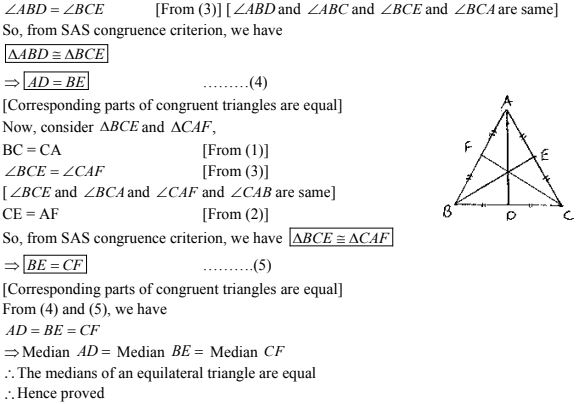5. In a ΔABC, if ∠A = 120° and AB = AC. Find ∠B and ∠C.
Solution
Consider a  ΔABC
Given that ∠A = 120 and AB = AC and given to find ∠B and ∠C
We can observe that ΔABC is an isosceles triangle since AB = AC
⇒ ∠B = ∠C  ...(1)
[Angles opposite to equal sides are equal]
We know that sum of angles in a triangle is equal to 180°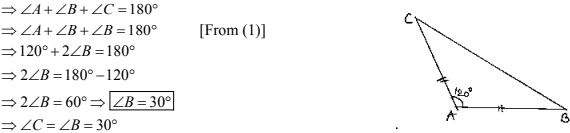6. In a ΔABC, if AB = AC and ∠B = 70° , find ∠A.
Solution
Consider ΔABC, we have ∠B = 70° and AB = AC
Since, AB = AC ΔABC is an isosceles triangle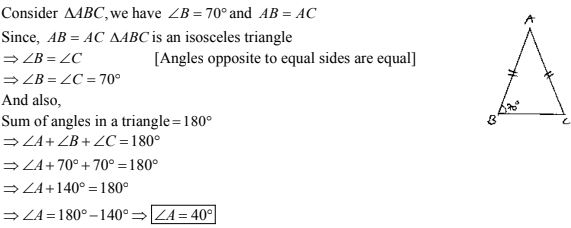7. The vertical angle of an isosceles triangle is 100  .Find its base angles.
Solution
Consider an isosceles  ΔABC such that AB = AC
Given that vertical angle A is 100 . Given to find the base angles
Since ΔABC is isosceles
∠B = ∠C  [Angles opposite to equal angles are equal]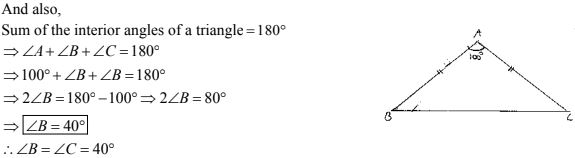8. In Fig, 10.24, AB  = AC and ∠ACD = 105, find ∠BAC.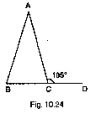Solution
Consider the given figure
We have,
AB = AC and ∠ACD = 105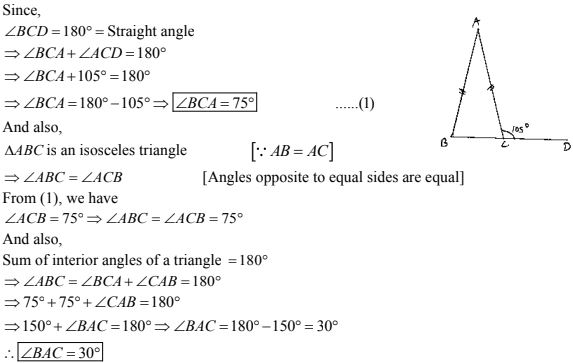9. Find the measure of each exterior angle of an equilateral triangle .
Solution
Given to find the measure of each exterior angle of an equilateral triangle consider an equilateral triangle ABC.
We know that for an equilateral triangle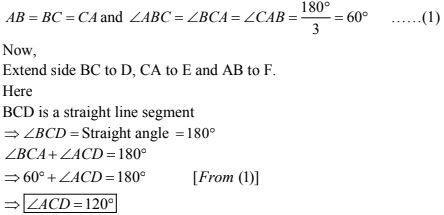Similarly, we can find ∠FAB and ∠FBC also as 120° because ABC is an equilateral triangle
∴ ∠ACD = ∠EAB = ∠FBC = 120°
Hence, the median of each exterior angle of an equilateral triangle is 120°

10. If the base of an isosceles triangle is produced on both sides, prove that the exterior angles so formed are equal to each other.
Solution
ED is a straight line segment and B and C are points on it.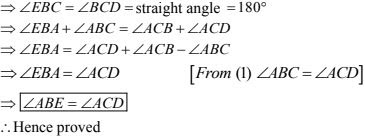11. In Fig. 10.25, AB = AC and DB = DC, find the ratio ∠ABD : ∠ACD.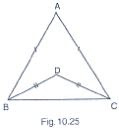Solution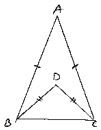Consider the figure
Given
AB = AC, DB = DC and given to find the ratio
∠ABD = ∠ACD
Now, ΔABC and ΔDBC are isosceles triangles since AB = AC and
DB = DC  respectively
⇒ ∠ABC = ∠ACB and ∠DBC = ∠DCB [∵ angles opposite to equal sides are equal]
Now consider,
∠ABD : ∠ACD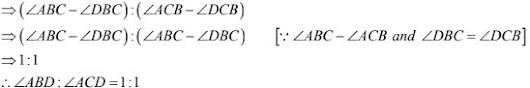12. Determine the measure of each of the equal angles of a right - angled isosceles triangle.
OR
ABC is a right - angled triangle in which ∠A = 90 and AB = AC. Find ∠B and ∠C.
Solution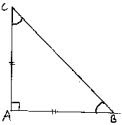Given to determine the measure of each of the equal angles of right angled isosceles triangle
Consider on a right - angled isosceles triangle ABC such that
∠A = 90° and AB = AC
Since, AB = AC ⇒ ∠C = ∠B  ...(1)
[Angles opposite to equal sides are equal]
Now,
Sum of angles in a triangle = 180°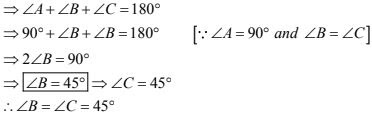Hence, the measure of each of the equal angles of a right - angled isosceles triangle is 45° .

13. AB is a line segment. P and Q are points on opposite sides of AB such that each of them is equidistant from the points A and B (See Fig. 10.26). Show that the line PQ is perpendicular bisector of AB.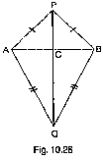Solution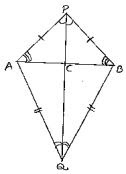Consider the figure,
We have
AB is a line segment and P, Q are points on opposite sides of AB such that
AP = BP  ...(1)
AQ = BQ  ...(2)
We have to prove that PQ is perpendicular bisector of AB.
Now consider ΔPAQ and ΔPBQ,
We have AP = BP   [∵ From (1)]
AQ = BQ  [∵ From (2)]
And PQ = PQ  [Common site]
⇒ΔPAQ ≅ ΔPBQ  ...(3) [From SSS congruence]
Now, we can observe that ΔAPB and ΔABQ are isosceles triangles. (From 1 and 2)
⇒ ∠PAB = ∠PBA and ∠QAB = ∠QBA
Now consider ΔPAC and ΔPBC,
C is the point of intersection of AB and PQ.
PA = PB   [From (1)]
∠APC = ∠BPC  [From (3)]
PC = PC  [Common side]
So, from SAS congruency of triangle ΔPAC ≅ ΔPBC
⇒ AC = CB and ∠PCA = ∠PCB  ...(4)
[∵ Corresponding parts of congruent triangles are equal]
And also, ACB is line segment
⇒ ∠ACP + ∠BCP = 180°
But ∠ACP = ∠PCB
⇒ ∠ACP = ∠PCB = 90°  ...(5)
We have AC = CB
⇒ C is the midpoint of AB
From (4) and (5)
We can conclude that PC is the perpendicular bisector of AB
Since C is a point on the line PQ, we can say that PQ is the perpendicular bisector of AB.